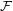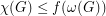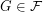Importance: Medium ✭✭
 Author(s): Geelen, Jim
 Subject: Graph Theory » Coloring » » Vertex coloring
 Keywords: chi-bounded circle graph coloring vertex minor
 Posted by: mdevos on: May 16th, 2009
Question   Is every proper vertex-minor closed class of graphs chi-bounded?

We say that a family of graphsis-bounded if there is a functionso thatfor every.

Ifis a simple graph, a vertex minor ofis any graph which can be obtained by a sequence of the following operations:

\item delete a vertex \item choose a vertexand complement the neighborhood of(i.e. wheneverare neighbors of, switchbetween adjacent/non-adjacent).

Dvorak and Kral [DK] showed that this conjecture is true for class of graphs of bounded rank-width, and the class of graphs having no vertex-minor isomorphic to the wheelonvertices.

## Bibliography

[DK] Z. Dvorak and D. Král. Classes of graphs with small rank decompositions are χ-bounded. European J. Combin., 33(4):679–-683, 2012. MathSciNet

* indicates original appearance(s) of problem.